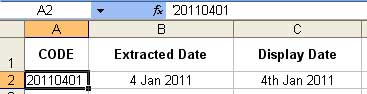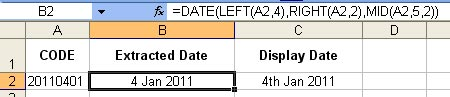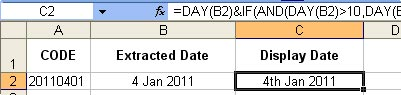# Fun With (Excel) Dates

I have been asked how to go about extracting the actual date from a code that is in  a date format.   The intended outcome will be as shown below:There are two important things to know.  One of them is that the code will always be entered in a consistent way.  The other is that you know which bit of the code is for the year, month and day (obviously).  In this example ‘20110401’ the first 4 digits represent the year, the next 2 digits are the day and the final 2 digits are the month.  It doesn’t matter whether the code is entered as a number or as text, the following solution will work.

The Excel functions required are the date function DATE and the text functions LEFT, RIGHT and MID.  I’ll go into the use of these in a future video but, to cut to the chase, the following image shows the formula required to extract the date from our code number.If you want to copy and paste this in to try it out then here you go: =DATE(LEFT(A2,4),RIGHT(A2,2),MID(A2,5,2))

If you are using Excel 2003 or above then, as you type in the formula, Excel will prompt you for the value it is expecting.  The order of entry is Year – Month – Day.   Once entered you can custom format the date any way you like.  If you want to know more about custom date formats see my YouTube tutorial on this topic.

Finally – and just to make life a little more complicated – supposing you wanted your days formatted as 1st, 2nd, 3rd instead of 1, 2, 3 etc?  Well here is the formula to do that (this is one way of doing it anyway)..In full that’s:

=DAY(B2)&IF(AND(DAY(B2)>10,DAY(B2)<20),”th”,CHOOSE(MOD(DAY(B2),10)+1,” th”,”st”,”nd”,”rd”,”th”,”th”,”th”,”th”,”th”,”th” ))&” “&TEXT(B2,”mmm”)&” “&TEXT(B2,”yyyy”)

The good news is that you only have to create the formula once and then simply copy down as many rows as you need.

Thanks to Mark for the question and I hope the solution is what you were looking for.  Have fun!

1.Monier says: